Back to the main index

# Multivariate pattern analysis with PyMVPA¶

Part of the introductory series Python for Vision Researchers brought to you by the GestaltReVision group (KU Leuven, Belgium).

In this part we discuss how to conduct multivariate pattern analysis on your fMRI data using PyMVPA, wrapped by the psychopy_ext.fmri module.

Author: Jonas Kubilius
Year: 2014
Copyright: Public Domain as in CC0

# Why use multivariate pattern analysis?¶

## Conventional (univariate) fMRI analysis¶

So you all know how a typical functional magnetic resonance imaging (fMRI) analysis goes like. You first collect some data, such as brain responses to faces, houses, objects, and bodies (as in Kanwisher et al., 1997), then use one half of the data to define a region of interest (ROI) using a particular contrast -- care about faces? good, take faces>objects -- then use the other half to check what it responds mostly to. (Why split data in half like this? Avoiding the double-dip issue here, see Kriegeskorte et al. (2009) for details.) If you chose the biggest blob in the fusiform gyrus as your ROI, you'll see that it responds twice as much to faces than to other stimuli. Congrats, you've got the fusiform face area (FFA) in your hands.

## Multivariate pattern analysis (MVPA)¶

OK, so you've got the FFA and now you want to understand the mechanisms governing responses in it. Does it respond only to full images of faces or perhaps two eyes and a nose will suffice to activate it? Or does it encode identity, gender, or race information? Great questions but it quickly becomes non-trivial to carry out such studies in practice. FFA responds robustly to faces as compared to non-faces, but differences in response to a male face versus a female face, if any, are likely to be tiny.

Moreover, it makes little sense for FFA to respond more to one gender than another. It is more likely that such differences are encoded by differences in activation of units within the FFA. For example, one population of neurons in the FFA might detect the presence of a male face, while another would encode the presence of a female face. On top of that, there are probably many units that respond to certain face features or their combinations irrespective to gender.

So if you carry out a conventional analysis, you will likely fail to find a significant difference even if it is actually encoded in the FFA. But notice that in a conventional analysis you are also throwing out all information conveyed in the pattern of response because you are averaging signal across all voxels within an ROI.

## Why does it work?¶

What exactly are we "decoding" with MVPA? Remember, voxels are not neurons -- there are hundreds of thousands of neurons within a voxel, all with their unique tuning properties. So rather than observing patterns of neuronal activation, all we have in each voxel is averages of these activations. Shouldn't we therefore get into the same problem as with univariate analyses?

There are two major theories here: hyperacuity and coarse scale biases (see Carlson, 2014).

Hyperacuity was proposed as a potential mechanism behind the success of MVPA. The idea is that in each voxel the distribution of neuronal preference is not uniform (see Boynton, 2005 for a nice illustration). When you take their average -- as happens in any given voxel -- some features will have a slightly higher response (because just by chance there are slightly more units preferring that feature). And that is what you end up picking up in MVPA. Given its origin in neural preferences, i.e., very small scale features, this theory is known as hyperacuity.

But we don't need these very fine scale anisotropies. An equally plausible idea is that features are grouping together into maps of a scale of several millimeters. So MVPA is simply picking up these large clusters of information.

To this day, there is a great deal of debate regarding the nature of signals that we are decoding. Moreover, can we really say we are decoding stimulus representations, or, similarly, can we claim that a particular area does not encode a particular feature if we fail to decode it? Of course not. When it works, MVPA informs us about the information available in that area but whether it represents anything or is merely epiphenomenal we cannot tell. Neurophychological or brain stimulation studies (TMS, optogenetics) might help to answer these questions. And when MVPA fails, then we ust don't know anything just like with any other null result studies.

# Explore the signal¶

## Setup¶

In this tutorial we will use the fmri module from the psychopy_ext package to do all the work. The fmri module provides a high-level interface to the PyMVPA package that was build specifically for MVPA analyses.

In the first step, we will generate synthetic fMRI data so that you don't have to download huge fMRI files. You will need approximately 341 MB space on your hard drive to store synthetized data.

In :
import numpy as np
np.random.seed(3)
from psychopy_ext import exp, fmri
PATHS = exp.set_paths(fmri_rel='%s')

fmri.GenHRF(PATHS).gen_test_data()

FreeType import Failed: expected string or Unicode object, NoneType found
Generating fMRI data... 1 2 3 4 5 6 7 8


## Data preprocessing¶

The first step is to preprocess your data. In SPM, we typicaly do the following steps:

1. Correct slice timing: correct differences in image acquisition time between slices because data during each TR is acquired sequentially from slices. Produces files with a prefix 'a'.
2. Realign images to the mean image because a participant is moving during scanning. Produces files with a prefix 'r'.
3. Estimate parameters of functional image coregistration to the anatomical volume so that we can compare the two and reconstruct 3D models of the brain.
4. Segment anatomical image into grey and white and cerebro-spinal fluid.
5. Normalize functional images to the MNI template using segmentation parameters. Produces files with a prefix 'w'.
6. Normalize anatomical image to the MNI template using segmentation parameters. Produces a file with a prefix 'w'.
7. Smooth functional images with a Gaussian kernel with full-width at half maximum (FWHM) being twice the voxel size. Produces files with a prefix 's'.

## Statistical model specification¶

Now we are ready to analyze data. We need to specify a statistical model for comparing brain responses to the conditions in the experiment. In SPM, it is the matter of providing the order of conditions in the experiment (produces beta-values) and specifying which conditions should be contrasted (produces t-values). It does so by fitting a hemodynamic response function (HRF) to the data. In general, beta-values tend to be more reliable but there's no guarrantee. These contrasts are useful for defining regions of interest (ROIs).

For non-localizer data analysis this step is not required but highly recommended as it produces better estimates of brain responses. However, in the rest of this course we will only use raw data, i.e., data as it comes out right after preprocessing.

## Region of interest (ROI) definition¶

ROIs can be defined in two ways at least:

1. By overlaying constrasts from the statistical model on top of your functional or anatomical data, and selecting the relevant voxels (typically, voxels that respond to one condition more than another above a certain threshold, like p < .0001 and requiring contiguous regions).

2. If the ROIs are small and easy to confuse with other ROIs (e.g., V1), 3D reconstruction of the brain is necessary. You can do so using Caret, FreeSurfer or many other packages. Once you have the 3D surface, it is much easier to define precisely the necessary ROIs.

In :
%matplotlib inline
from psychopy_ext import exp, fmri
PATHS = exp.set_paths(fmri_rel='%s')

mvpa = fmri.Analysis(PATHS, 2, info={'subjid':'subj_01', 'runtype':'main'}, rois='rh_LO')
mvpa.plot_roi(roi='rh_LO.nii')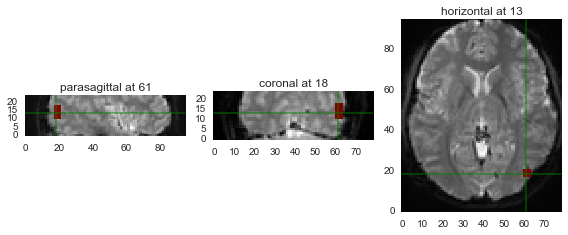## Detrending¶

Once images and ROIs are ready, we can inspect brain responses in them. We extract functional data for each ROI but before we look into the signal, we need to detrend it.

Throughout a scan session, signal intensity tends to increase for a variety of reasons (and that is one reason why you should always use palindromic stimuli sequences in blocked designs). This increase is not meaningful at all so we need to detrend it -- that is, find this global tendency of increasing responses and subtract it. By default, detrending in psychopy_ext module is done using a second order polynomial for each run separately.

## Timecourse of the signal¶

We are finally ready for having the first peak into the data by ploting timecourse of the signal. In psychopy_ext, you need to specify in the rp parameter that you want to visualize data which will plot the full timecourse of the first run, as shown in the example below:

In :
%matplotlib inline
from psychopy_ext import exp, fmri
PATHS = exp.set_paths(fmri_rel='%s')

mvpa = fmri.Analysis(PATHS, 2, info={'subjid':'subj_01', 'runtype':'main'},
rp={'values':'raw', 'method':None, 'visualize':True},
rois='rh_LO', dur=4, offset=0)

subj_01 rh_LO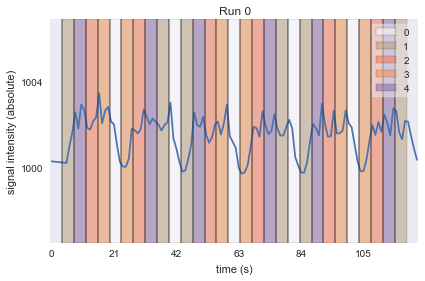An exception has occurred, use %tb to see the full traceback.

SystemExit

To exit: use 'exit', 'quit', or Ctrl-D.


You can also plot the average signal timecourse over the entire experiment for each condition:

In :
%matplotlib inline
from psychopy_ext import exp, fmri
PATHS = exp.set_paths(fmri_rel='%s')

mvpa = fmri.Analysis(PATHS, 2, info={'subjid':'subj_01', 'runtype':'main'},
rp={'values':'raw', 'method':'timecourse', 'force':'all'},
rois='rh_LO', dur=8, offset=0)

 subj_01 rh_LO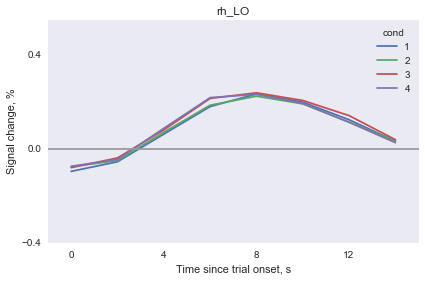# Correlational analyses¶

The most straightforward way to run MVPA is to compute a correlation between two patterns of response within an ROI. In particular, you can split your data in half and compute pairwise correlations between all conditions. If this correlation between the same condition is higher than the correlation between different conditions, then we say that information about conditions is available to that brain area. (For a very nice and simple study using this analysis, see Williams et al., 2007.)

Let's go over the steps implementing this analysis in practice.

Let's plot responses of each voxel in an ROI (columns) for the whole run (rows) to get a feeling of what they are like:

In :
%matplotlib inline
from psychopy_ext import exp, fmri
import matplotlib.pyplot as plt
import seaborn as sns

sns.set(style='dark')
PATHS = exp.set_paths(fmri_rel='%s')

mvpa = fmri.Analysis(PATHS, 2, info={'subjid':'subj_01', 'runtype':'main'},
rp={'values':'raw', 'method':None, 'force':'all'},
rois='rh_LO', dur=2, offset=2)
ds = mvpa.extract_samples('subj_01', 'main', ['rh_LO.nii', 'rh_LO', '*rh_LO.nii'],
values='raw', offset=2)
ds = mvpa.detrend(ds)
evds = mvpa.ds2evds(ds, dur=2)
mvpa.plot_ds(ds)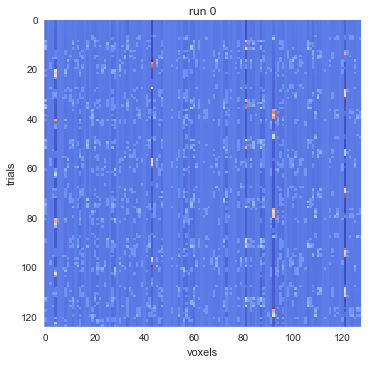## Normalization and averaging¶

Before we use these data, it is important to normalize neural responses. It is possible that for one condition responses are generally higher than to another, thus we might be leveraging these global biases. Typically, data is normalized by subtracting the mean across conditions per run per voxel.

Moreover, we should average data across trials.

In :
import mvpa2.suite
import numpy as np

# leave fixation out
evds = evds[evds.sa.targets != mvpa.fix]

# calculate the mean per target per chunk (across trials)
run_averager = mvpa2.suite.mean_group_sample(['targets','chunks'])
evds_avg = evds.get_mapped(run_averager)
numt = len(evds_avg.UT)

# calculate mean across conditions per chunk per voxel
target_averager = mvpa2.suite.mean_group_sample(['chunks'])
mean = evds_avg.get_mapped(target_averager)
# subtract the mean chunk-wise
evds_avg.samples -= np.repeat(mean, numt, 0)


## Training and testing sets¶

Alright, so we're finally ready to do some real MVPA! We are now going to split data in half and correlate one half with another. Remember: if there is no information about conditions in the response patterns, then it is effectively random and everything will correlate with everything to the same extent. However, if there is some information, we should see a higher correlation (lower dissimilarity) between patterns of response corresponding to the same condition than between patterns of response corresponding to different conditions.

In :
targets = evds_avg.UT

# split 1
evds_split1 = evds_avg[:4]
run_averager = mvpa2.suite.mean_group_sample(['targets'])
evds_split1 = evds_split1.get_mapped(run_averager)

# split 2
evds_split2 = evds_avg[4:]
run_averager = mvpa2.suite.mean_group_sample(['targets'])
evds_split2 = evds_split2.get_mapped(run_averager)

result = mvpa2.clfs.distance.one_minus_correlation(evds_split1.samples,
evds_split2.samples) / 2

plt.imshow(result, cmap='coolwarm', interpolation='none')
plt.colorbar()
plt.show()

# plot averages for within-condition and across-conditions
within = result.trace() / result.shape
across = (np.sum(result) - result.trace()) / (result.size - result.shape)
ax = plt.subplot(111)
ax.bar(0, within, label='within')
ax.bar(1, across, label='across')
ax.legend()
plt.show()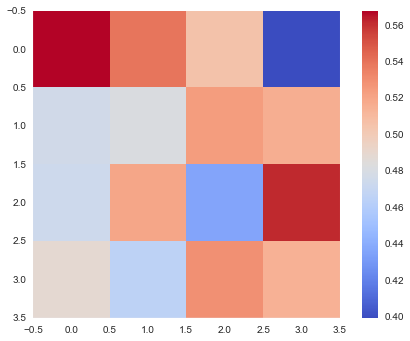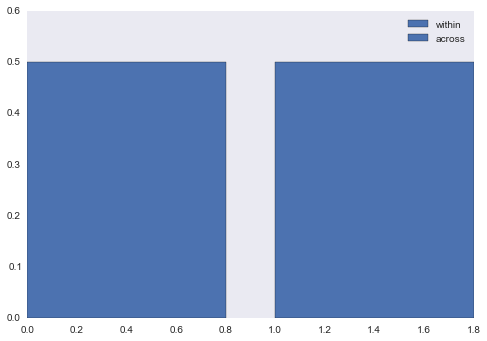## Cross-validation¶

So there seems to be something in the pattern of response. Before we celebrate, there is an extra step recommended here. Did you notice how we split the data? We put the first four runs in split1 and the remaining for in split2. Why split like that? Couldn't we have chosen some other kind of split?

We could and, in fact, we should. Because if we don't, how can we know that the result is not spurious, that is, that the observed difference is not due the particular split that we performed? Maybe another split would not yield the same result? And, on the other hand, we might completely miss the effect because just by accident it was not present in the particular split that we chose. Of course, in practice we have several participants so if the effect is stable across participants, we are doing fine. But chance are higher to observe the effect if we average outcomes of several splitting procedures. In psychopy_ext, by default random splitting is performed 100 times. We will refer to this procedure as cross-validation though the true meaning of this term will become clear only when we consider support vector machines.

In :
%matplotlib inline
from psychopy_ext import exp, fmri
PATHS = exp.set_paths(fmri_rel='%s')

mvpa = fmri.Analysis(PATHS, 2, info={'subjid':'subj_01', 'runtype':'main'},
rp={'values':'raw', 'method':'corr', 'plot':True},
rois='rh_LO', dur=2, offset=2)

subj_01
Could not load or find the results file ./analysis/corr_raw_subj_01.pkl
Will proceed to do corr analysis from scratch
rh_LO
C:\Python27\lib\site-packages\numpy\core\_methods.py:79: RuntimeWarning: Degrees of freedom <= 0 for slice
warnings.warn("Degrees of freedom <= 0 for slice", RuntimeWarning)
C:\Python27\lib\site-packages\matplotlib\figure.py:1595: UserWarning: This figure includes Axes that are not compatible with tight_layout, so its results might be incorrect.
warnings.warn("This figure includes Axes that are not "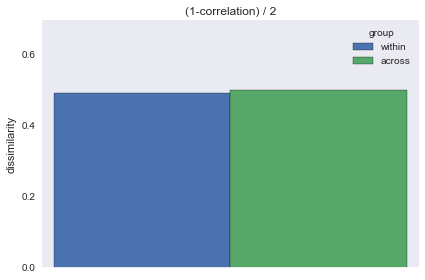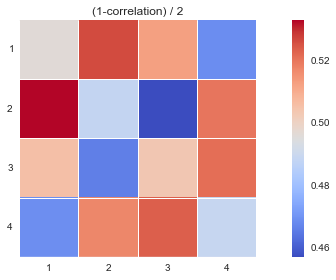# Support vector machines¶

## Introduction¶

Correlational analyses are simple and fast but they might have issues dealing with noisy fMRI data. In the analysis we just did, it is really not so clear it there is anything or not. This is the major problem of interest in machine learning so here we will employ one of their most robust and powerful techniques, known as support vector machines (SVM). (Note that in practice SVMs are not guarranteed to yield a better result that correlations.)

## Setup¶

In :
"""
Modified from PyMVPA example http://www.pymvpa.org/examples/pylab_2d.html
and http://www.pymvpa.org/examples/pylab_2d.html
"""
import mvpa2.suite
import numpy as np
import matplotlib.pyplot as plt
import seaborn
%matplotlib inline

np.random.seed(3)

##############
# Parameters #
##############
npoints = 200
xymax = 9  # Absolute max value allowed. Just to assure proper plots
ngrid = 101  # grid for evaluting performance

# set grid
x1 = np.linspace(0, xymax, ngrid)
x2 = np.linspace(0, xymax, ngrid)
x,y = np.meshgrid(x1, x2)
feat_grid = np.array((np.ravel(x), np.ravel(y)))

def train_svm(ds, cl= mvpa2.suite.LinearNuSVMC):
# define classifier
clf = cl(probability=1, enable_ca=['probabilities'])
# enable saving of the estimates used for the prediction
clf.ca.enable('estimates')
# train with the known points
clf.train(ds)
# run the predictions on the test values
pre = clf.predict(feat_grid.T)
# get probabilities from the svm
res = np.asarray([(q - q + 1) / 2
for q in clf.ca.probabilities])
# get support vectors
supvecs = clf.model.get_sv()

return res, supvecs

def plot_data(ds, supvecs=None, contour=None):
ax = plt.subplot(111)
ax.set_aspect('equal')

# plot samples
ax.plot(ds.samples[ds.targets == 1, 0], ds.samples[ds.targets == 1, 1], '.',
color='indianred', label='condition 1')
ax.plot(ds.samples[ds.targets == 0, 0], ds.samples[ds.targets == 0, 1], '.',
color='steelblue', label='condition 2')

# plot support vectors as larger dots
if supvecs is not None:
feat_pos = ds.samples[ds.targets == 1]
feat_neg = ds.samples[ds.targets == 0]
pos_test = np.array([f for f in feat_pos if f in supvecs]).T
neg_test = np.array([f for f in feat_neg if f in supvecs]).T
ax.plot(pos_test, pos_test,
'o', color='indianred')
ax.plot(neg_test, neg_test,
'o', color='steelblue')

# plot probability contour
if contour is not None:
# plot decision surfaces at few levels to emphasize the topology
z = np.asarray(contour).reshape((ngrid, ngrid))

ax.contour(x, y, z, [0.1, 0.4, 0.5, 0.6, 0.9],
linestyles=['dotted', 'dashed', 'solid', 'dashed', 'dotted'],
linewidths=1, colors='black', hold=True)

ax.set_xlabel('response in voxel 1')
ax.set_ylabel('response in voxel 2')
ax.legend()


## Data separation problem¶

The basic idea here is that for each condition we have the data as n-dimentional vectors where n is the number of voxels and we want to know if those vectors are somehow separable. In other words, we want to find a particular partitioning rule that would allow us to predict with confidence from which condition new data points would be. If we can do so, we can claim that in that there is information about those conditions in the given ROI.

To illustrate this, let's consider responses from just two voxels (so that's a 2-dimentional space that we can plot). The responses are, of course, all over the place but it is not so difficult to decide which response belongs to which category. What do you think the optimal separation would be?

In :
#################
# Generate data #
#################
dist = 1  # distance between the two distributions

def gen_data(dist):
feat = mvpa2.suite.pure_multivariate_signal(30, 2)
feat = np.random.randn(2, npoints) + dist + xymax / 2.
feat = feat.clip(0, xymax)
return feat

# two categories of samples
feat_pos = gen_data(dist)
feat_neg = gen_data(-dist)

# create the pymvpa dataset from the labeled features
pat_pos = mvpa2.suite.dataset_wizard(samples=feat_pos.T, targets=1)
pat_neg = mvpa2.suite.dataset_wizard(samples=feat_neg.T, targets=0)
ds = mvpa2.suite.vstack((pat_pos, pat_neg))

# plot data
plot_data(ds)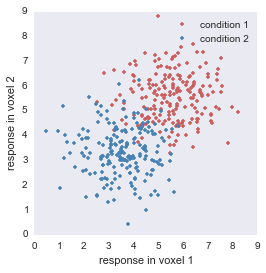Here is what SVM thinks an optimal separation is (look at the black solid line; other lines indicate decreasing probabilities of where the boundary could be):

In :
# train SVM and plot
res, supvecs = train_svm(ds)
plot_data(ds, supvecs=supvecs, contour=res)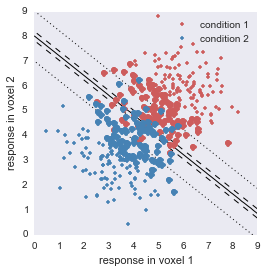Well, that looks pretty good. I mean, it could have provided many other solutions where this separation line could be, such as slightly rotated lines. But in fact, SVM is guarranteed to find the optimal separation boundary and this is why this technique is so great.

It is also fast. One of the reasons it is fast is that it detects the so-called support vectors, i.e., the data points that are close to the separation boundary, and uses only them to make it decision. Everything else doesn't matter so we can use even very large datasets.

It is also important to understand that SVMs are not magic that can do everything by themselves. They have certain parameters that a user can manipulate in order to yield better results. By default, in PyMVPA these parameters are set to some hopefully reasonable values and we do not manipulate them. It might be better to perform a grid-search for best parameters but for some reason this is not done in practice.

## Is that the true separation boundary?¶

Of course not. This is the optimal boundary given the data. The true boundary, in this case, should be a line at a 45 deg angle. But SVM cannot know the underlying distribution absolutely from just several hundred of samples. Need more precision? Get more data.

## SVM on our data¶

OK, that's all you realy need to know about SVMs. Time to test them on our data!

Procedure:

• Normalize data by subtracting the mean across voxels per chunk per condition (target).
• Split data into a training set (about 75% of all values) and a testing set (about 25% of values), unless there are only two runs, in which case it is 50% training and 50% testing.
• For each pair of conditions, train the classifier (this is a binary classification; multiclass SVM is also available but I don't use them).
• Then test on the average of the testing set, i.e., only on two samples. This trick usually boosts the performance (credit: Hans P. Op de Beeck)
In :
%matplotlib inline
import numpy as np
np.random.seed(3)
from psychopy_ext import exp, fmri
PATHS = exp.set_paths(fmri_rel='%s')

mvpa = fmri.Analysis(PATHS, 2, info={'subjid':'subj_01', 'runtype':'main'},
rp={'values':'raw', 'method':'svm', 'plot':True},
rois='rh_LO', dur=2, offset=2)

subj_01
Could not load or find the results file ./analysis/svm_raw_subj_01.pkl
Will proceed to do svm analysis from scratch
99 %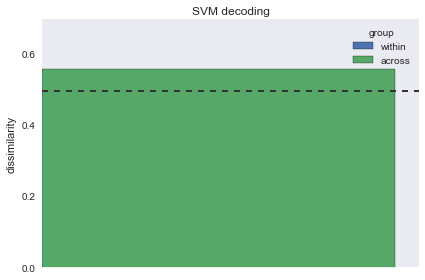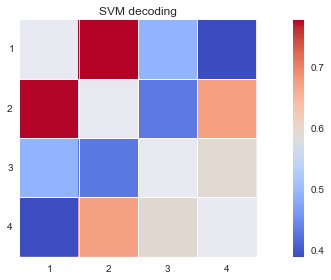## Training and testing sets¶

Notice -- and this is very important -- that we always split data into two sets, and one of them is used for training only, while the other is used for testing only. This way, we train SVM on one data set and then, using another set, ask how well it learned the separation between conditions. What do you think its performance would be if we trained and tested on the same dataset?

Answer: usually you would get a very good performance, not 100%, but good nonetheless because for most datasets SVM will be able to find some sort of a separating hyperplane that does indeed separate the two conditions to some extent. However, the whole point is to see if this hyperplane is actually useful at all in general, not just for this training set. That's why we use a separate testing set to check whether there is a general separation rule.

## Cross-validation¶

So what is this cross-validation? In psychopy_ext, we split data randomly into training and test samples. If we did it in a more systematic manner this would yield the cross-validation. For example, a popular cross-validation technique is know as leave-one-out where all but one runs are used in the training set and the remaining one is the testing set. In our case, we would take the first run as the testing set and the remaining seven runs as the training set. In the next iteration, we would take the second run as the testing run and the other seven as the training set. And so forth. In the end, this would be an eight-fold cross-validation as if we folded the data in eight different ways.

Importantly, data always remains independent.

## Nonlinear classifiers¶

So far we only used linear classifiers in our examples, i.e., those that are only capable of drawing a hyperplane between two classes. But what if the distributions of the signal look like this:

In :
ds = mvpa2.suite.pure_multivariate_signal(npoints, 2)
ds.samples += 6
plot_data(ds)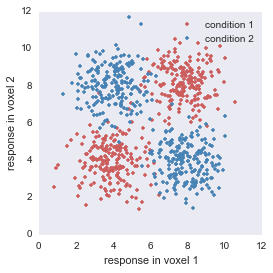There is no way you can separate these two populations linearly. But you can clearly see a difference between two populiations so surely there must be a way to do that. And surely there is because this is a very common situation that machine learning has to deal with. Instead of using linear classifiers we can simply use their nonlinear variants. In the example below, we use an SVM with the radial basis function kernel.

In :
res, supvecs = train_svm(ds, cl=mvpa2.suite.RbfNuSVMC)
plot_data(ds, supvecs=supvecs, contour=res)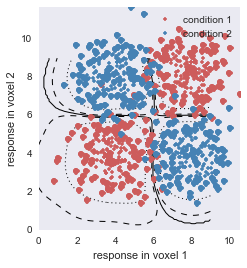Awesome! Nonlinear classifiers works amazingly in machine learning. But should you use this in neuroimaging? Probably not. To illustrate that, imagine taking images with and without faces. You can go ahead and train your nonlinear classifier on these images or, if you want to relate it to brain responses, first filter them with Gabor-line filters just like in V1. The classifier will likely be able to differentiate between the two categories -- but will you want to make a claim that V1 does face processing? From what we know, V1 does not detect face information. Yet we can decode faces from it. Why?

Well, the information about faces present or absent is in the signal (the image) itself by definition, so given a powerful enough model I will find this difference. But it does not mean that V1 makes use of this information. For its purposes, this information is unusable. The whole point of visual processing is to deconvolve signals into something usable, something easily discriminable. In neuroscience, we tend to assume that a linear separation is easy enough for the next layer in the visual hierarchy to perform.

Not everybody necessarily agrees with this resctriction though.